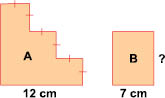# User Forum

Subject :NCO    Class : Class 4

Figure A and B have the same perimeter. Find the width of figure B.(a) 12 cm
(b) 8 cm
(c) 17 cm
(d) 18 cm

## Ans 1:

Class : Class 4
Oh I understand now.EXPLANATION IS Let's start with fig. A. Both of the long lines are of the same length though one of them may seem the same. Now going to the short lines. Divide 12 by 3. Now multiply by 6. Jot down your answer. It should be 48. Now fig. B. It is a rectangle. You know the formula for the perimeter of a rectangle right? Now do these calculations 7 into 2. Ans 14. Now 14 ? Divided by 2= 48?Ans is 17

## Ans 2:

Class : Class 4
THIS IS THE MOST TOUGH QUESTION

Class : Class 4